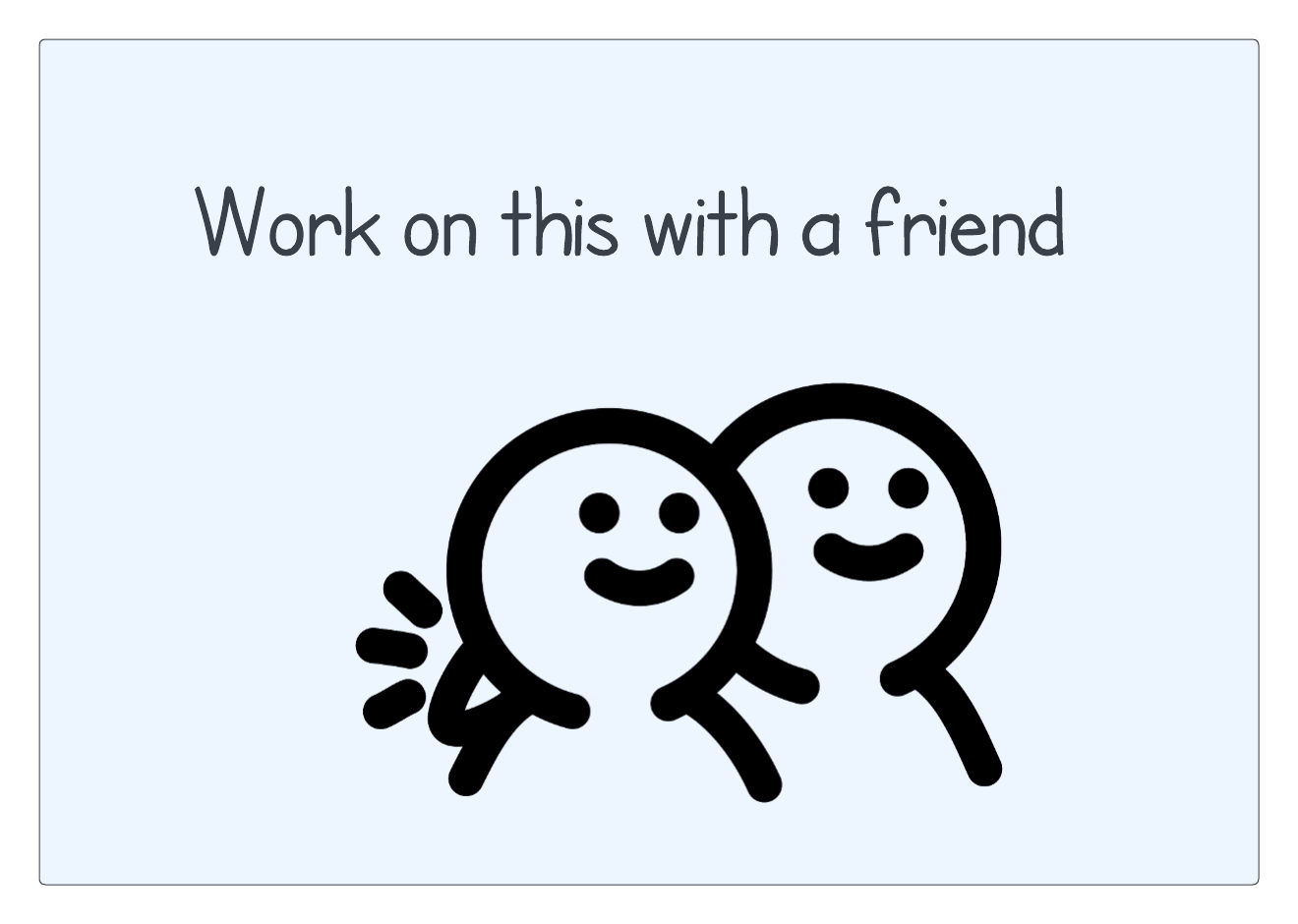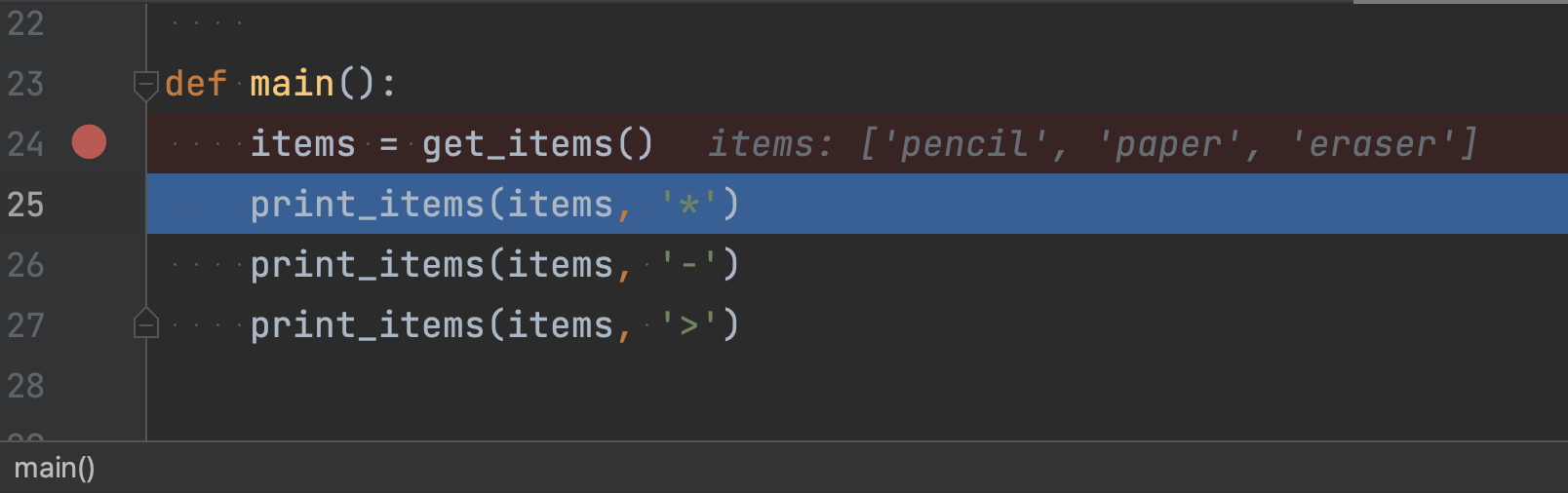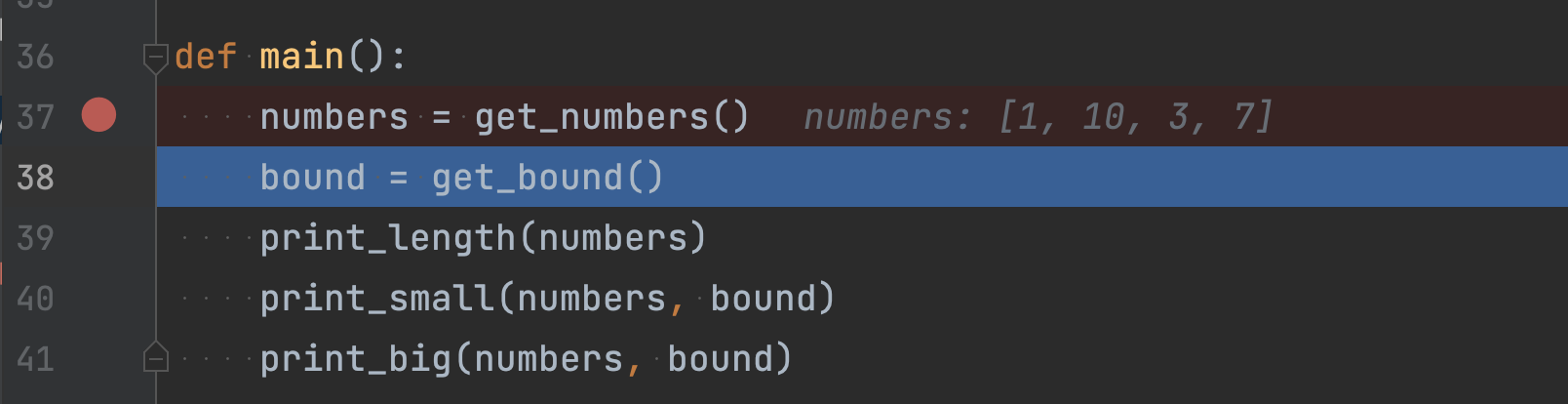Computer Science

# Practice with lists

We are going to write several programs to practice using lists.

## Bullets styles

Write a program that:

• Asks a person for a series of items.
• The person inputs `q` when they are done.
• Prints the list of items using the following bullets:
• `*`
• `-`
• `>`

So the list of items is printed three times, each time using a different type of bullet.

### Planning

See if you can write this program with a friend. You have starter code in the zip file above, in the file called `bullet_styles.py`:

``````def main():
# Write code here
pass

if __name__ == '__main__':
main()``````

Start by decomposing the problem into functions! What are the functions you would use in `main()`?### Basic solution

There are two parts to this problem:

• getting the list of items
• printing the list of items, with a certain kind of bullet

These translate directly into two functions:

``````def main():
items = get_items()
print_items(items, '*')
print_items(items, '-')
print_items(items, '>')``````
• `get_items()` takes no parameters — it should get the entire list of items and return a list

• `print_items()` takes two parameters — (1) the list, and (2) a string with the type of bullet to use

This is how you should think as you write programs — decompose the problem into functions first, and then write the functions one at a time.

### Getting the items

We can write an empty function for `print_items()`:

``````def print_items(items, bullet):
pass``````

This lets us focus on writing `get_items()`:

``````def get_items():
"""
Prompt the user for items until they provide 'q'
Return the items in a list
"""
items = []
while True:
item = input('Item: ')
if item == 'q':
break
items.append(item)

return items``````
• loop forever
• add each item to the list
• if the person enters `q` then break from the loop
• return the list, which now has all the items the person entered

If you run this program, you can see something like this:

``````Item: pencil
Item: paper
Item: eraser
Item: q``````

It seems to be working but how do we know if `get_items()` has returned a list properly? There are a couple of things you can do. First, you can use `print()`:

``````def main():
items = get_items()
print(items)
print_items(items, '*')
print_items(items, '-')
print_items(items, '>')``````

Now if you run the program, you see:

``````Item: pencil
Item: paper
Item: eraser
Item: q
['pencil', 'paper', 'eraser']``````

That’s probably enough to convince us that this is working just fine! But we have to remember to remove your `print()` statements when we are done.

Another option is to use the debugger. Put a breakpoint in `main()` on the line that calls `get_items()`. Then run the debugger and step over the `get_items()` function. After you enter your items using the console, you should see:This is a good way to get used to the debugger, so we recommend doing this instead of using `print()`.

### Printing the items

Now we can write the `print_items()` function:

``````def print_items(items, bullet):
"""
Print a list of items using the provided bullet character.
Print an extra blank line at the end of the list.
"""
for item in items:
print(f'{bullet} {item}')
print()``````
• `print_items()` takes two arguments, the list to print and a bullet character.
• We iterate through the list using `for ... in`
• We use string formatting to print the list items
• We use `print()` by itself to print a blank line

Now if you run the code, you should see something like this:

``````Item: pencil
Item: paper
Item: eraser
Item: q
* pencil
* paper
* eraser

- pencil
- paper
- eraser

> pencil
> paper
> eraser
``````

Great!

## Big and small

Write a program that asks a person for a list of numbers (one number at a time).Then ask the user what number to use as the boundary between “big” and “small” numbers. The program should then print:

• You have [how_many] numbers
• These are small:
• [all of the small numbers, one per line]
• These are big:
• [all of the big numbers, one per line]

### Planning

See if you can write this program with a friend. You have starter code in the zip file above, in the file called `big_and_small.py`:

``````def main():
# Write code here
pass

if __name__ == '__main__':
main()``````

Notice that this is the same starter code as the previous problem. You should eventually get used to typing this in yourself.

Start by decomposing the problem into functions! What are the functions you would use in `main()`?### Basic solution

For this problem, we have a number of things to do:

• getting the list of numbers
• getting the boundary number
• printing the number of numbers
• printing the small numbers
• printing the big numbers

These translate directly into two functions:

``````def main():
numbers = get_numbers()
bound = get_bound()
print_length(numbers)
print_small(numbers, bound)
print_big(numbers, bound)``````

We can create empty functions for each of these:

``````def get_numbers():
pass

def get_bound():
pass

def print_length(numbers):
pass

def print_small(numbers, bound):
pass

def print_big(numbers, bound):
pass``````

This lets us write and test one function at a time.

### Getting the numbers

We can write the `get_numbers()` function using the same input loop as above when we got items:

``````def get_numbers():
numbers = []
while True:
response = input('Number: ')
if response == 'q':
break

number = int(response)
numbers.append(number)

return numbers``````

Notice an important addition — we need to convert the person’s entry into an integer using `int()`.

You should be able to test this with the debugger:### Getting the boundary number

We can write the `get_bound()` function:

``````def get_bound():
return int(input('Boundary: '))``````

Note that we are getting the input, converting it to an integer, and returning it all in one step. This code does the same thing:

``````def get_bound():
response = input('Boundary: ')
return int(response)``````

and so does this code:

``````def get_bound():
response = input('Boundary: ')
integer_response = int(response)
return integer_response``````

Whether you do it in multiple steps or one is up to you!

You can use the debugger to see if this is working:### Printing the information

Now we can fill out the remaining functions, which do some printing:

``````def print_length(numbers):
print(f'You have {len(numbers)} numbers')

def print_small(numbers, bound):
print('These are small:')
for number in numbers:
if number < bound:
print(number)

def print_big(numbers, bound):
print('These are big:')
for number in numbers:
if number >= bound:
print(number)``````
• `print_length()` is just one line and uses a formatted string
• `print_small()` and `print_big()` both use `for ... in` to iterate over the numbers and print them out

If you run the program, you will see something like this:

``````Number: 10
Number: 1
Number: 3
Number: 7
Number: q
Boundary: 5
You have 4 numbers
These are small:
1
3
These are big:
10
7``````